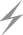Computer Aids for VLSI Design
Steven M. Rubin
Copyright © 1994

# Appendix E: EBES Format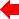Appendix 5 of 7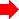One of the most popular formats for producing integrated-circuit masks is the Electron Beam Exposure System (EBES), which can specify the very small features needed in high-density chips. Although the actual format varies with each different company's mask-making machine, an original standard, designed at Bell Laboratories, forms the basis on which extensions are made. This basic format is described here.

The electron beam is controlled in a digital raster fashion, such that a line of 256 points (called address units) can be written at a time. By convention, this sweep is run vertically and the mask is moved horizontally to make the sweep cover the top 256 rows of the chip (see Fig. E.1). It thus takes multiple passes across the width of the chip to write a complete pattern. When multiple copies of an IC die are being produced on a wafer, a single sweep of 256 rows is made on every die position before advancing to the next 256 rows. This means that the pattern file, which is organized in 256 row stripes, needs to be read only once.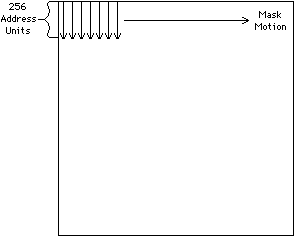FIGURE E.1 EBES raster motion. Actual mask making sweeps out 256 rows as it advances horizontally.

## E.1 File Structure

The EBES file is organized about these 256 row stripes. The beginning of the file contains a START DRAWING command followed by the stripes and an END DRAWING command. Each stripe consists of a START STRIPE command, a series of figure commands, and an END STRIPE command. The figures can be either RECTANGLEs, PARALLELOGRAMs, or TRAPEZOIDs.

EBES files are binary, with 16 bits per word. Commands can take any number of words but must be aggregated into 1024-word blocks. If a command is near the end of a block and would span into the next block, then an END OF BLOCK command must appear followed by pad data to the block end. All blocks must end with the END OF BLOCK command except for the last block, which ends with the END DRAWING command.

## E.2 Control Commands

The START DRAWING command is the first in an EBES file and contains 16 words. The first word has a 2 in the high byte and a code in the low byte that gives the size of an address unit. The address-unit size will be 1 micron if the code is 0, one-half micron if the code is 3, and one-quarter micron if the code is 6. The second and third words of the START DRAWING command are the x and y size of the entire pattern. Words 4, 5, and 6 contain the EBES file creation date: Word 4 is the month, word 5 is the day, and word 6 is the year. For these fields, the high byte is the first digit and the low byte is the second digit in ASCII. Words 7 through 13 contain the name of this file by having a name length in word 7 (always the value 6) and by having the next six words (8 to 13) contain an ASCII string with the format "XXXXXXXXX.XX". Finally, words 14 through 16 describe the current mask layer by having word 14 contain a 2 and words 15 and 16 contain four ASCII characters.

After the START DRAWING command comes the first START STRIPE command. This command is one word with the value 7 in the low byte and the stripe number (from 1 to 255) in the high byte. Note that the word and byte sizes form restrictive limits on the overall pattern size. For larger dies to be made, multiple pattern files must be abutted.

After the START STRIPE come the figures. When all figures in a stripe have been listed, an END STRIPE command appears. The END STRIPE is a single word with the value 8. At the end of a block is an END OF BLOCK command, which is a single word with the value 9. At the end of the EBES file is an END DRAWING command, which is a single word with the value 4.

## E.3 Rectangles

The RECTANGLE is one of the figures allowed in a stripe. It contains four words that describe the width, height, and corner position within the stripe. The first word contains the value 16 in the low 6 bits and contains the rectangle height (minus one) in the high 10 bits. Since the rectangle must fit in a 256-tall stripe, this height can have only the values 0 to 255. The second word of the rectangle command is the width, the third word is the starting x position, and the fourth word is the starting y position. Again, the y position can range only from 0 to 255 since it must be within the stripe.

## E.4 Parallelograms

The PARALLELOGRAM command is similar to the RECTANGLE command except that it has two more words to give a skew distance and extra precision bits (see Fig. E.2). Word 1 has the value 17 in the low 6 bits and a value that is one less than the height in the top 10 bits. Words 2, 3 and 4 define the width, x position, and y position as in the RECTANGLE command, except words 2 and 3 are multiplied by 16. Word 5 contains the x offset between the bottom and the top (also multiplied by 16). The sixth word contains extra precision bits for the width (the low 5 bits), the x location (the next 5 bits), and the x offset (the high 6 bits). These extra precision bits are high bits for the three horizontal position values, and define 21- or 22-bit fields for each. The reason for this extra precision is not to extend the addressing range but to allow fractional coordinates: These three values are in 1/16 address units. Thus there is an implied decimal point 4 bits from the right on all these numbers.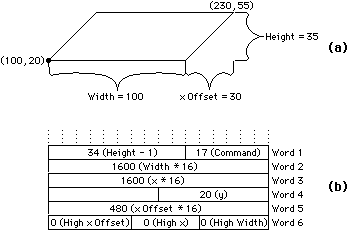FIGURE E.2 EBES parallelogram example: (a) Figure (b) Record.

## E.5 Trapezoids

There are three types of TRAPEZOIDs, as illustrated by Fig. E.3. Type 1 has a vertical right edge, type 2 has a vertical left edge, and type 3 has no vertical edges. The type 1 and 2 TRAPEZOIDs can be described in exactly the same format as is used for the PARALLELOGRAM because they have the same fields: x, y, width, height, and x offset. Type 1 TRAPEZOIDs have the value 18 in the low 6 bits of word 1, and type 2 TRAPEZOIDs have the value 19 in this field.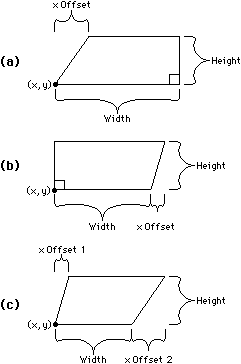FIGURE E.3 EBES trapezoid types: (a) Type 1 has vertical edge on right (b) Type 2 has vertical edge on left (c) Type 3 has no vertical edges.

Type 3 TRAPEZOIDs require a seven-word description because of the extra x offset field. Word 1 has a 20 in the low 6 bits and the height minus one in the top 10 bits. Word 2 is the width, word 3 is the x location, word 4 is the y location, word 5 is the first x offset, word 6 is the second x offset, and word 7 contains additional precision bits for the width (low 5 bits), the x location (next 5 bits), and the first x offset (top 6 bits). The extra precision bits for the second x offset are in word 4 (the top 6 bits), which is otherwise used only for its low 8 bits to contain the y location.Previous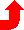Table of Contents NextSteven M. Rubin Static Free Software